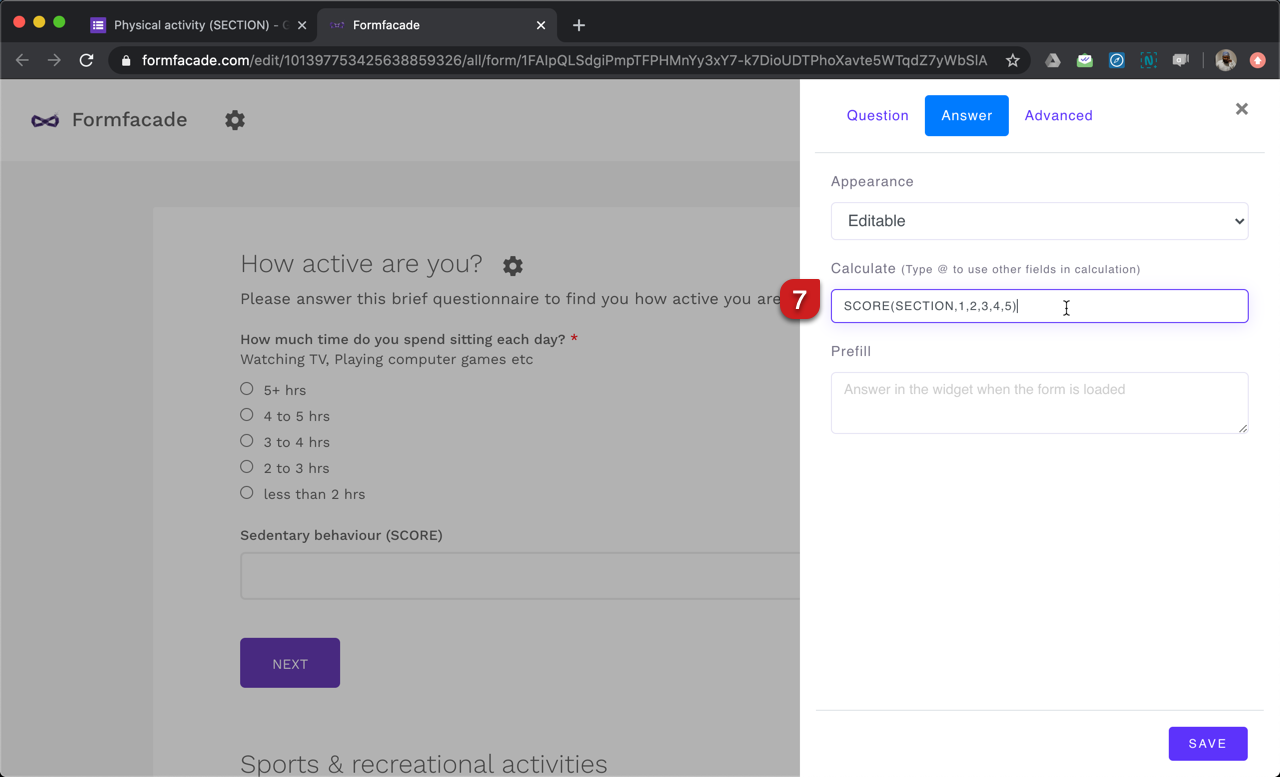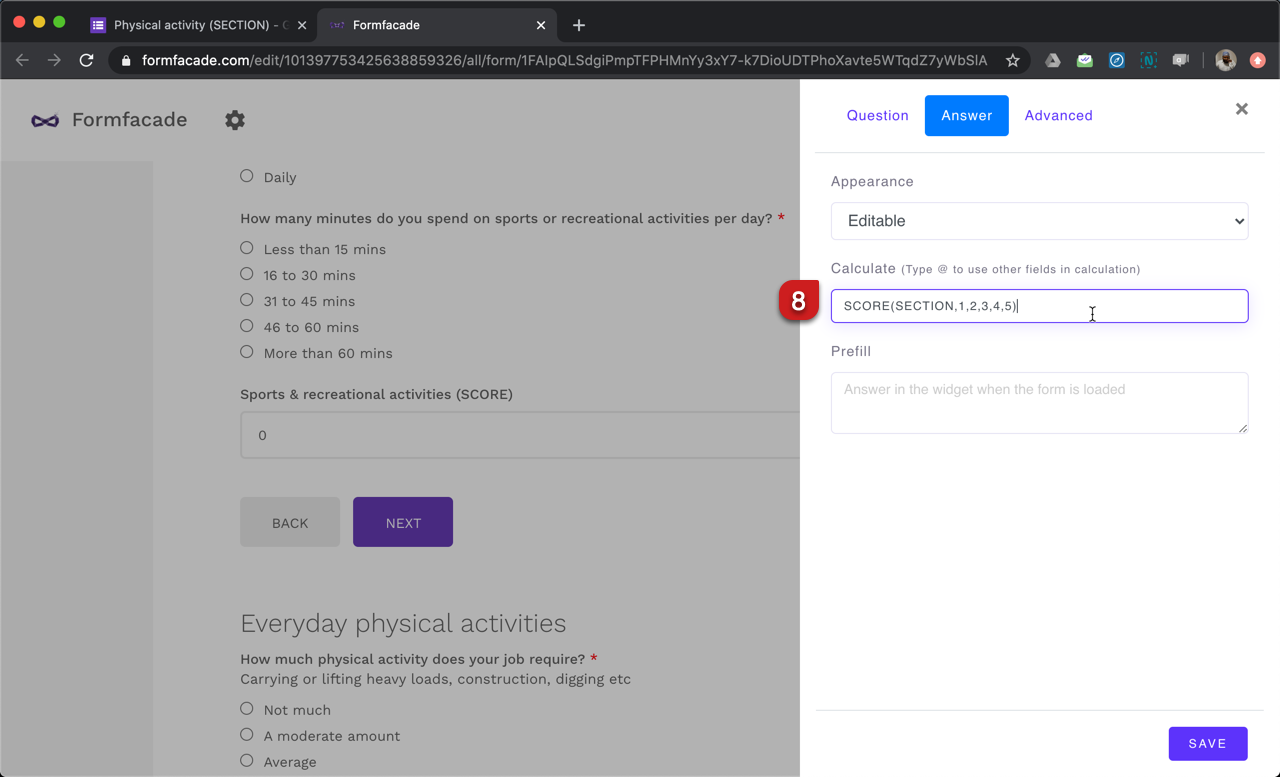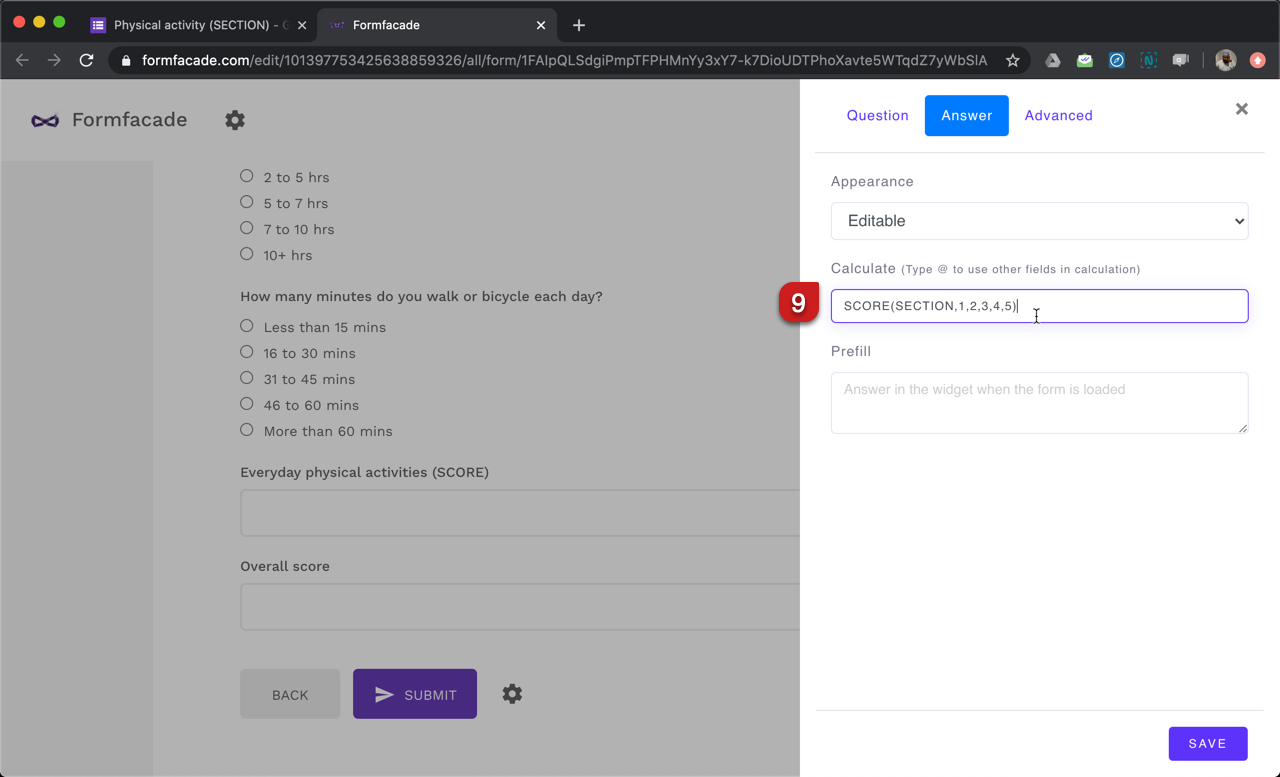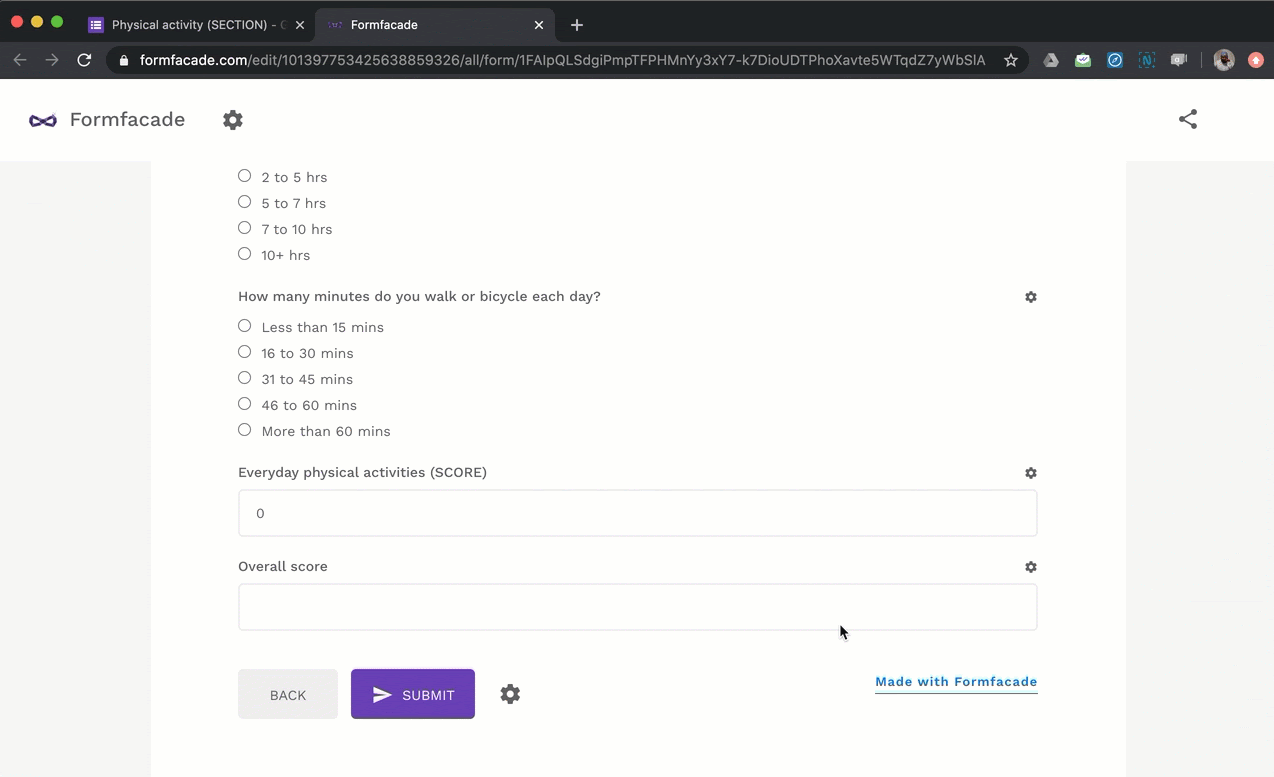### You can assign point values to questions in Google Forms by creating a quiz and calculate the total score, but you cannot calculate the individual scores for each section. To do that, you can use the Formfacade addon for Google Forms.#### How to calculate individual scores for each section using Formfacade?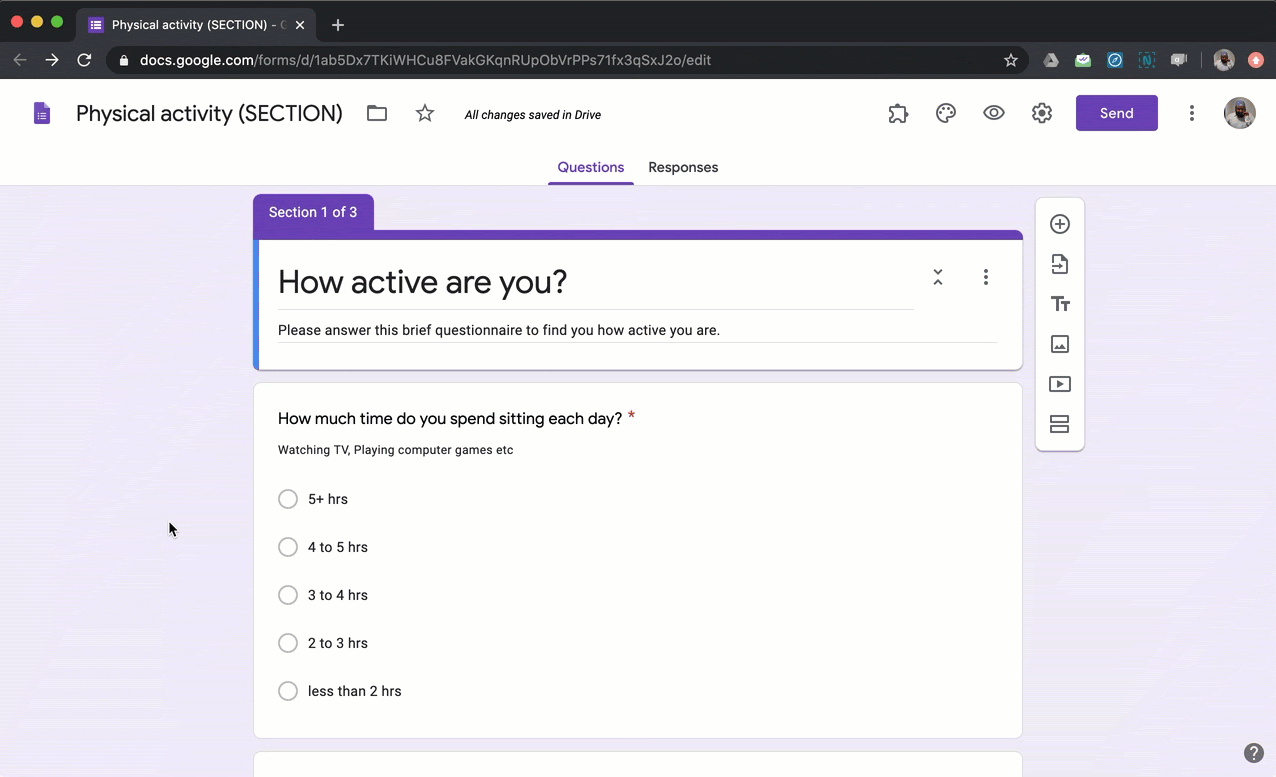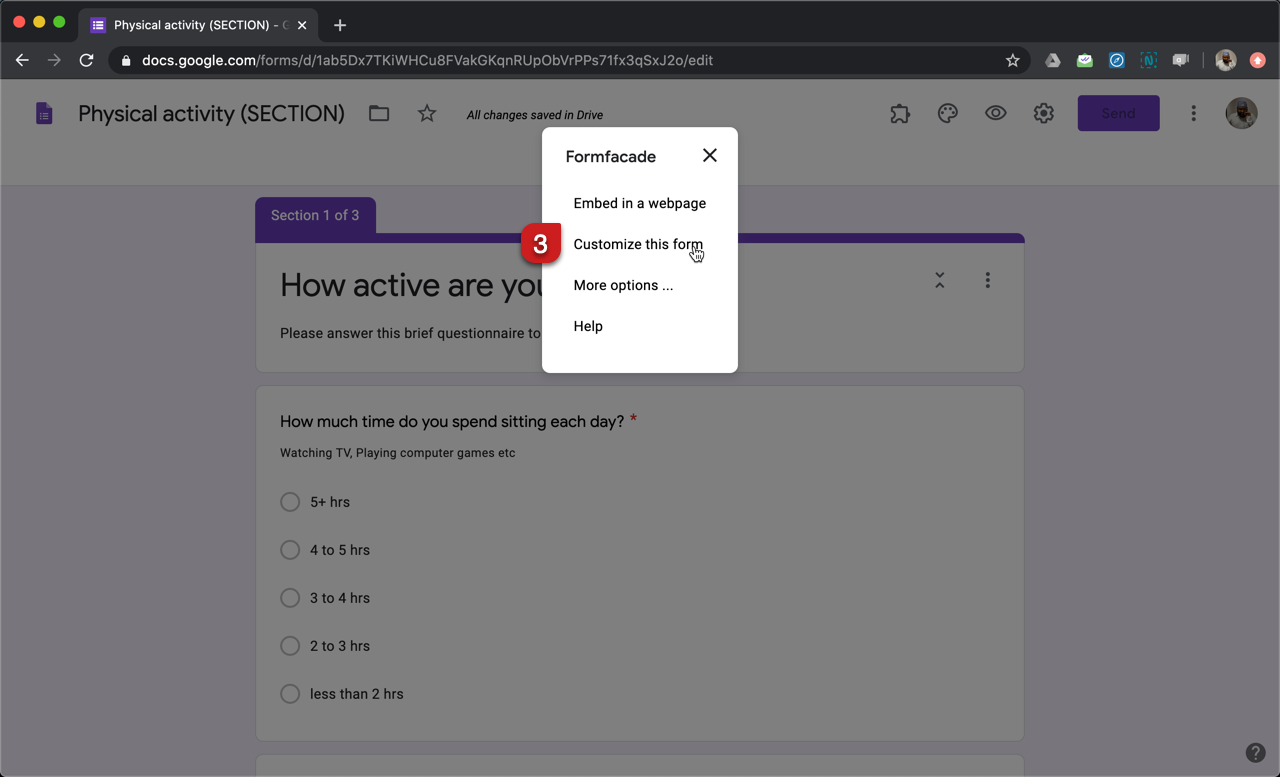#### SCORE function

SCORE() function allows you to assign scores to each answer choices in multiple choice questions, checkboxes questions and grid questions. It takes two input parameters:
1. The first parameter specifies whether the score should be calculated for all questions in the section or questions across all the sections or only the questions that matches the search term in the description.
2. The second parameter is the points to be assigned for different answer choices (a), (b), (c), (d), (e) etc.

In this example, we will use SCORE function to calculate the individual scores for the three sections Sedentary behaviour, Sports & recreational activities and Everyday physical activities.

SCORE(SECTION,1,2,3,4,5)
First parameter: SECTION option to calculate the score for questions in the current section.
Second parameter: 1, 2, 3, 4, 5 to assign points for answer choices (a), (b), (c), (d), (e) respectively for all the questions in the current section.